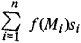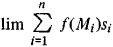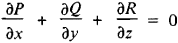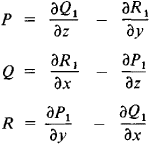Surface Integral

Also found in: Wikipedia.

surface integral

[′sər·fəs ′int·ə·grəl]
(mathematics)
The integral of a function of several variables with respect to surface area over a surface in the domain of the function.
McGraw-Hill Dictionary of Scientific & Technical Terms, 6E, Copyright © 2003 by The McGraw-Hill Companies, Inc.
The following article is from The Great Soviet Encyclopedia (1979). It might be outdated or ideologically biased.

Surface Integral

the integral of a function defined on some surface. For example, the problem of calculating the mass distributed over a surface S with a variable surface density f(M) leads to a surface integral. To calculate this mass, we divide the surface into parts with areas s1, s2, …, sn, and select in the ith part a point Mi. If these parts are sufficiently small, their masses are approximately equal to f(Mi)si, and the mass of the entire surface is approximately equal toThe smaller the parts si, the more exact is this value. The exact value of the mass of the surface is thereforeif the dimensions and the areas of the parts approach zero. Certain problems in physics result in similar limits. These limits are called surface integrals of the first kind for the function f(M) with respect to a surface S and are symbolized

∫∫(s) f(M) ds = ∫∫(s) f(x, y, z)ds

The evaluation of such integrals reduces to the calculation of double integrals.

Limits of similar sums are also encountered in other problems in physics, for example, in determining the flow of a fluid across a surface S. In these problems, however, the areas of the projections of the parts on the three coordinate planes are used instead of the areas of the parts. Here S is assumed to be oriented— that is, one of the directions of the normals is indicated as positive; the area of the projection is assigned a plus or minus sign, depending on whether the angle between the positive direction of the normal and the axis perpendicular to the plane of the projections is acute or obtuse. The limits of sums of this type are surface integrals over oriented surfaces, and are called surface integrals of the second kind and are symbolized

∫∫(s) P dy dz + Q dz dx + R dx dy

In contrast to surface integrals of the first kind, the sign of surface integrals of the second kind depends on the orientation of the surface S.

M. V. Ostrogradskii established an important theorem that gives the relation between the surface integral of the second kind over a closed oriented surface S and the triple integral over the volume Vbounded by S. It follows from this theorem that if the functions P, Q, and R have continuous partial derivatives and the equationis satisfied in V, then the surface integrals of the second kind over all oriented surfaces contained in V and having the same boundary are equal. In this case, functions P1, Q1, and R1 can be found such thatStokes’ theorem expresses the line integral over a closed curve in terms of the surface integral of the second kind over an oriented surface bounded by this curve.

REFERENCE

Nikol’skii, S. M. Kurs matematicheskogo analiza, vol. 2. Moscow, 1973.
Il’in, V. A., and E. G. Pozniak. Osnovy matematicheskogo analiza, part 2. Moscow, 1973.
Kudriavtsev, L. D. Matematicheskii analiz, 2nd ed. vol. 2. Moscow, 1973.
References in periodicals archive ?
To alleviate this problem, a spherical harmonics expansion-based MLFMA (SE-MLFMA) for the dyadic form of surface integral equation (SIE) was proposed .
Using a continuous approach, atoms are assumed to be evenly distributed over the molecules and the average atomic densities provided are averaged over its surface, which means that the summation over all atoms involved is replaced by surface integrals. The magnitude of total energy arising from molecular interatomic energy is then obtained mathematically by performing a surface integral over the surface of each molecule, given by
The surface integral equation (SIE) method is a powerful tool for their electrodynamic analysis [1,2].
In this method, the fields are expanded in terms of wave functions and the field expansions are substituted in the surface integral equations equivalent to the Helmholtz equation with the standard boundary conditions.
Following this, the description of the hybrid finite element method/boundary element method (FEM/BEM) approach  and the surface integral equation (SIE) approach , respectively, are given.
A damage index DI(x, y) is defined as surface integral of Hilbert-Huang spectrum on these featured regions as follows:
The time average wall shear stress (TAWSS) and its surface integral at bifurcation sites were quantified.
Again, we employ Gauss' theorem to express the total energy as a surface integral:
This linearity allows the application of the surface integral equation method or the boundary element method (BEM) which maybe more efficient than the boundary value methods such as the finite element method (FEM) or the finite difference method (FDM).
Actually, the method of moments (MoM) solutions of surface integral equations (SIEs) are widely used for numerical analysis of EM radiation and scattering problems [1-9].
Being compared with the surface integral equation method, the FDTD approach is more effective for modeling inhomogeneous objects and complex geometries.
Determination of mechanical work Wet of elastic deformation is made by correlating it with the surface integral [S.sub.i] of the loading curve:

Site: Follow: Share:
Open / Close